﻿ Dynamic Analysis of a Dual-Disk Rotor Bearing System with Parametric Excitations

### Dynamic Analysis of a Dual-Disk Rotor Bearing System with Parametric Excitations

BSN Murthy, J. Srinivas, Ravi Pratap Singh, K. Udaya Sri, LSNVP KiranOPEN ACCESSPEER-REVIEWED

## Dynamic Analysis of a Dual-Disk Rotor Bearing System with Parametric Excitations

BSN Murthy1, J. Srinivas2, Ravi Pratap Singh2,, K. Udaya Sri3, LSNVP Kiran1

1Mechanical Engineering Department, GITAM University, Visakhapatnam, India

2Mechanical Engineering Department, NIT, Rourkela, India

3Mechanical Engineering Department, KG Reddy College of Engg. & Technology, Hyderabad, India

### Abstract

This paper deals the dynamic analysis of a flexible low-speed rotor model having both rotational asymmetries and transverse shaft cracks. Asymmetric disks or transverse shaft cracks in rotors lead to parametric inertia (or stiffness) excitations in rotor-bearing system. When both of them appear in a rotor system, prediction of parametric instability behavior has not gained sufficient attention. Disk asymmetry is considered in terms of the mass and damping terms and shaft stiffness is dictated by the transverse crack location and depth. The rotor is discretized into five elements and the resultant double periodic problem is solved using explicit Runge-Kutta time integration scheme. The specialty of the problem is that in every time step of integration, an assembled stiffness and mass matrix is formulated. The resulting five coupled nonlinear second-order system of equations are solved and the unbalance response of the rotor is obtained under various conditions of relative disk asymmetries and crack depth ratio. The present outcomes can be employed for identification of the rotor system from the vibration response.

### At a glance: Figures

1234
Prev Next

• Murthy, BSN, et al. "Dynamic Analysis of a Dual-Disk Rotor Bearing System with Parametric Excitations." Journal of Mechanical Design and Vibration 2.4 (2014): 81-86.
• Murthy, B. , Srinivas, J. , Singh, R. P. , Sri, K. U. , & Kiran, L. (2014). Dynamic Analysis of a Dual-Disk Rotor Bearing System with Parametric Excitations. Journal of Mechanical Design and Vibration, 2(4), 81-86.
• Murthy, BSN, J. Srinivas, Ravi Pratap Singh, K. Udaya Sri, and LSNVP Kiran. "Dynamic Analysis of a Dual-Disk Rotor Bearing System with Parametric Excitations." Journal of Mechanical Design and Vibration 2, no. 4 (2014): 81-86.

 Import into BibTeX Import into EndNote Import into RefMan Import into RefWorks

### 1. Introduction

In rotating systems, there are several time variable loads acting at the bearings, disks as well as on the shaft system. These may be due to the nonlinear characteristics of ball and roller bearings, internal damping, cracks on the shaft under radial clearance and so on. As the rotating speed increases, these nonlinearities have vital influence on dynamic characteristics. Therefore, accurate identification of the model structure and parameters of rotor bearing system incorporating such nonlinear effects is of utmost importance. Compared to parametric estimation, identification of nonlinear model structure is a complex issue. There are various other types of nonlinearities involved in rotors such as geometric stiffening effect, self-excited vibrations due to internal friction, forced oscillations of rotor with distributed masses, material nonlinearities, internal damping mechanism etc. Likewise, parametric instabilities are crucial in the design and usage of rotor bearing systems. Most of the time parametric instability is caused by transverse cracks on the shafts. When the system has simultaneous nonlinearities due to transverse cracks on shaft and disk asymmetries, the dynamic characteristics of the system are tedious to compute. Several authors studied the dynamics of rotors subjected to different types of parametric excitations and various techniques were proposed to identify the nonlinearities in the system. Using linear models, comprehensive methodology for fault identiﬁcation of unbalance were studied [eg., [1, 2]]. Gaetan  reviewed some of the popular approaches in system identiﬁcation of nonlinear dynamical structures. Shiyu and Jianjun  presented an on-line estimation method that can simultaneously estimate the parameters and determine the signiﬁcance of the nonlinear and time variant effects in the rotor-bearing system, based on an order down dating algorithm. D’Sauza and Epureanu  proposed a generalized minimum rank perturbation theory for identifying damage in nonlinear systems. Coulomb friction and cubic stiffness nonlinearity have been considered. Platten et al.  presented the identification method for large nonlinear systems based on the use of a multi-exciter arrangement with the restoring force surface method. Luke et al.  highlighted the inadequacy of linear-based methodology in handling initially nonlinear systems and also shown how the recently developed autoregressive support vector machine approach can be used for detecting damage in a system that exhibits initial nonlinear response. Patel and Darpe  investigated the inﬂuence of the crack breathing models on the nonlinear vibration characteristics of the cracked rotors. Redmond  presented a model which enables dynamic analysis ﬂexibly coupled misaligned shafts.

Often, the disk on rotors have inertial asymmetries as in two-blade propeller, fan and pumps, wind turbine etc. There is unequal rotary inertia about two principal axes of the rotating disk resulting in unknown dynamics. Asymmetry can cause large vibrations due to parametric resonances. Recently several authors [10, 11, 12] modelled the dynamics of inertial asymmetric systems. Transverse cracks in rotors also cause the parametric instability and enormous literature [e.g., [13-17]] is available on nonlinear dynamic stability analysis of shafts with transverse cracks. Identification of such asymmetry and transverse crack in rotor from the dynamic response characteristics is of crucial importance in modern engineering technology. Present work concentrates first on the analysis of a rotor model with disk inertial asymmetry (changes in mass) and shaft transverse crack (reduction in stiffness). A two rigid disk geometric model is accounted with flexible supports. Disk asymmetry is considered in terms of the mass and damping changes while, the transverse crack (location and depth) alters the stiffness of the rotor. Dynamic equations are formulated and solved using time integration scheme. The unbalance response of the rotor is obtained at different conditions of asymmetric angles and geometry and depth of transverse crack. An inverse model is developed using neural networks to predict disk asymmetry and crack depth using dynamic response amplitudes and frequencies.

### 2. Mathematical Modelling

Present model of rotor consists of a shaft system carrying two disk as shown in Figure 1, supported over flexible bearings.

The shaft is considered to have a transverse crack at some location and it is assumed that, both the disks have asymmetries. The asymmetric disk has four degrees of freedom, including two translations of mass center and two rotations. Therefore, it’s nodal displacement vector is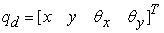. The mass of the disk is md. Three moments of inertia about the z-axis, x-axis and y-axis (respectively one polar and two diametral) are represented by Ip, Ix and Iy. For the inertia asymmetry, Ix ≠ Iy. The constant rotational speed of the rotor is denoted by. The formulation of the disk in the fixed coordinates is written as :

Where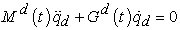(1)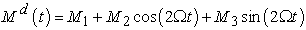(2)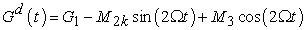(3)

Here,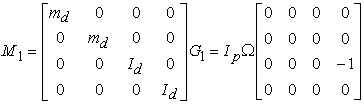(4)(5)

In which Id= (Idx+Idy)/2 is the mean value of the diametral moments of inertia andis the relative inertia asymmetry of the disk varying from 0 to 1. This indicates, the mass and damping of disk are sinusoidal time periodic with frequency twice the rotational speed. The shaft crack is assumed at angle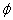relative to the shaft coordinate axes at a time t=0 as shown in Figure 2.

As the shaft rotates, the crack angle changes with the negative Y-axis to. The stress field only changes in the region adjacent to the crack. The additional strain energy for a crack facilitates in writing the stiffness matrix. For the open crack, there are two factors that lead to the time-varying moments of inertia of cross-sections: one is that the area moments of inertia of the cracked element about the X and Y have time-periodic values as the shaft rotates and other is that the coordinates of the centroid relative to the X and Y axes are also time-variable. The open-cracked element stiffness matrix can be written in a generalized form as: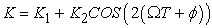(6)

Where the matrices K1 and K2 are given by(7)

Here,are constants and I1, I2 are given as: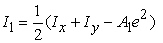(8a)(8b)

Further, Ix, Iy and A1 are:(9)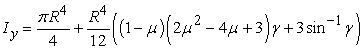(10)(11)

With, relative crack depthandas dimensionless parameters. Here, R is shaft radius and h is the crack depth.

2.1. Resultant Finite Element Model

FEM model of rotor system: the assembled finite element matrices of the shaft and disk are considered and the resultant equations of the motion are written as follows: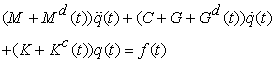(12)

Here, M, C, G and K are the matrices corresponding to shaft obtained as an assemblage of beam elements accounting rotary inertia and shear deformation , while Md(t) and Gd(t) refers to the secondary matrices of zero entries except for the disk nodes and Kc(t) is invariable stiffness matrix of zero entries except for cracked element. Also, f(t) is vector of unbalance and gravity forces. The system of equations reduces to second order time-varying periodic differential equations with frequency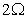. Writing y(t) =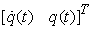, equation (12) reduces to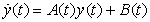(13)

In which(14)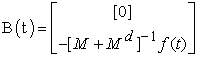(15)

### 3. Results and Discussion

The present rotor system is analyzed using Runga-Kutta constant time step method. A user interactive program is developed in MATLAB with five elements. Here, an unbalance eccentricity of 60 µm is selected on both the disks. The element-4 is considered to have a crack. At every time step, the program computes the overall stiffness and mass matrices and generates the time history, orbit-map and frequency response at required nodes. The values of the physical parameters are given in Table 1.

#### Table 1. Important physical parameters of rotor-bearing-disk system usedDownload asPowerPoint Slide

Veiw figureView current table in a new window

Figure 3 shows the time histories at disk-1 node. All the data is taken at=0.1 and speed N=1000 rpm. It is observed that there is no marked effect ofon the time response. Figure 4 shows the whirl orbits and Figure 5 shows the corresponding FFT plot at disk 1. It is seen that the amplitude in the first mode is larger for=0.6 in FFT diagram indicating the effect of crack depth on modal amplitude.

Figure 3. Time histories at disk 1 for=0 and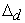=0.1
Figure 4. Whirl Orbit plot at disk 1 for=0 and=0.1
Figure 5. Frequency response at disk 1 for=0 and=0.1
Figure 6. Frequency response at disk 1 for=90 degree and=0.1

Figure 6 shows the frequency response at two different values ofcorresponding to=90. There is a marked change in the amplitudes when compared with=0. Finally Figure 7 shows the effect ofat constant values ofand.

Figure 7. Effect of asymmetry at=90 degree and)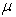=0.3

It is also observed that beyond=0.4, the program fails to give meaningful result indicating that it would not be a physically possible disk condition.

3.1. Inverse Approach of Prediction of Crack State

As it is obvious that the frequency response is affected by all these 3 parameters:,, and, in addition to the crack location and speed of rotor, we can develop an inverse approach using an optimization scheme for estimating the crack and asymmetric parameters. In present task, the first two natural frequencies from frequency response are employed in formulating objective function. The methodology is explained in the Figure 8.

The optimization scheme has to be selected (GA or MATLAB toolbox) for this minimization process.

3.2. Experimental Methodology

The double disk rotor system is arranged as shown in the Figure 8 and rotor shaft is made to run with D.C. motor at various speeds using variable frequency drive (VFD).

The Laser Doppler vibrometer (LDV-100) is used to take the response data and analyse the data with Polytec Vibrometer software (VibSoft) for required information. Figure 9 shows the experimental set-up usded for measurements.

Figure 10 shows the time domain response obtained at the mid length of the shaft at 2500 rpm and Figure 11 represents the corresponding FFT plot at node 3. It is seen that the approximate natural frequency as around 200 Hz.

Figure 12 shows an equivalent plot obtained from computer simulations of original rotor without considering disk asymmetry and shaft crack

The maximum peak in the response correspond to a value close to 200 Hz. It is also necessary to compare the affected rotor response using both experimentally and numerically. The work is under progress.

### 4. Conclusions

Influence of shaft crack and asymmetric disk parameters on the overall frequency response of a dual disk rotor supported on flexible bearings has been studied in this paper. Even though model is standard, the user interactive computer program developed in Matlab helps to assess the vibration response at different locations along the rotor length. It is observed that lower crack depth maintains stability in the system. Such a parametric instability should be studied at higher speeds. As a future scope inverse methodology for prediction of disk asymmetry and crack parameters from dynamic response may be attempted using neural networks and fuzzy logic systems.

### References

  N.D.S. Sudhakar and A.S. Sekhar, “Identiﬁcation of unbalance in a rotor bearing system”, Journal of Sound and Vibration, vol. 330, pp. 2299-2313, 2011.In article CrossRef  M.B. Deepthikumar, A .S. Sekhar and M.R. Srikanthan, “Modal Balancing of flexible rotors with bow and distributed unbalance”, Journal of Sound and Vibration, vol. 332, pp. 6216-6233, 2013.In article CrossRef  K.Gaetan, K.Worden, A.F. Vakakis and J.C.Golinval, “Past, Present and future of nonlinear system identiﬁcation in structural dynamics”, Journal of Mechanical Systems and Signal Processing, vol. 20, pp. 505-592, 2006.In article CrossRef  Z.Shiyu and S.Jianjun, “Identiﬁcation of non-linear effects in rotor systems using recursive QR factorization method”, Journal of Sound and Vibration, vol. 270, pp. 455-469, 2004.In article CrossRef  K.D’Souza and B.I.Epureanu, “Multiple augmentations of nonlinear systems and generalized minimum rank perturbations for damage detection”, J. Sound and Vibration, vol. 23, pp. 101-121, 2008.In article CrossRef  M.F. Platten, J.R. Wright, G. Dimitriadis, and J.E. Cooper, “Identification of multi-degree of freedom nonlinear systems using an extended modal space model”, Mech. Systems and Signal Processing, vol. 23, pp. 8-29, 2009.In article CrossRef  B.Luke, R.Charles and P. Gyuhae, “Damage detection in initially nonlinear systems”, International Journal of Engineering Science, vol. 48, pp. 909-920, 2010.In article CrossRef  T. H. Patel and A.K. Darpe, “Inﬂuence of crack breathing model on nonlinear dynamics of a cracked rotor”, Journal of Sound and Vibration, vol. 311, pp. 953-972, 2008.In article CrossRef  I. Redmond, “Study of a misaligned ﬂexibly coupled shaft system having nonlinear bearings and cyclic coupling stiffness-Theoretical model and analysis”, Journal of Sound and Vibration, vol. 29, pp. 700-720, 2010.In article CrossRef  Q.Han and F.Chu, “The effect of transverse crack upon parametric instability of a rotor-bearing system with an asymmetric disk”, Comm. Nonlinear Science & Numerical Simulations, vol. 17, pp. 5189-5200, 2012.In article CrossRef  Q.Han and F.Chu, “Parametric instability of a Jeffcott rotor with rotationally asymmetric inertia and transverse crack”, Nonlinear Dynamics, vol. 73, pp. 827-842, 2013.In article CrossRef  I.Bucher and O.Shomer, “Asymmetry identification in rigid bodies-Theory and Experiment”, Mech. Systems and Signal Processing, vol.41, pp. 502-509, 2013.In article CrossRef  L.M.Dai and C.P.Chen, “Dynamic stability analysis of a cracked nonlinear rotor system subjected to periodic excitations in machining”, Journal of Vibration and Control, vol. 13 (5), pp. 537-556, 2007.In article CrossRef  C.P.Chen, L.M.Dai and Y.M.Fu, “Nonlinear response and dynamic stability of a cracked rotor”, Communication in Nonlinear Science and Numerical Simulation, vol.12, pp. 1023-1037, 2007.In article CrossRef  J.Sinou, “Effects of a crack on the stability of a non-linear rotor system”, International Journal of Non-Linear Mechanics, vol. 42 (7), pp. 959-72, 2007.In article CrossRef  R.T.Liong and C.Proppe, “Application of the cohesive zone model for the evaluation of stiffness losses in a rotor with a transverse breathing crack”, Journal of Sound and Vibrations, vol. 332, pp. 2098-2110, 2013.In article CrossRef  M.Silani, S.Z.Rad and H.Talebi, “Vibration analysis of rotating systems with open and breathing cracks”, Applied Mathematical modeling, vol. 37, pp.9907-9921, 2013In article CrossRef  M.Lalanne and G. Ferraris, Rotor dynamics prediction in engineering. 2nd ed. New York: John Wiley & Sons; 1990.In article